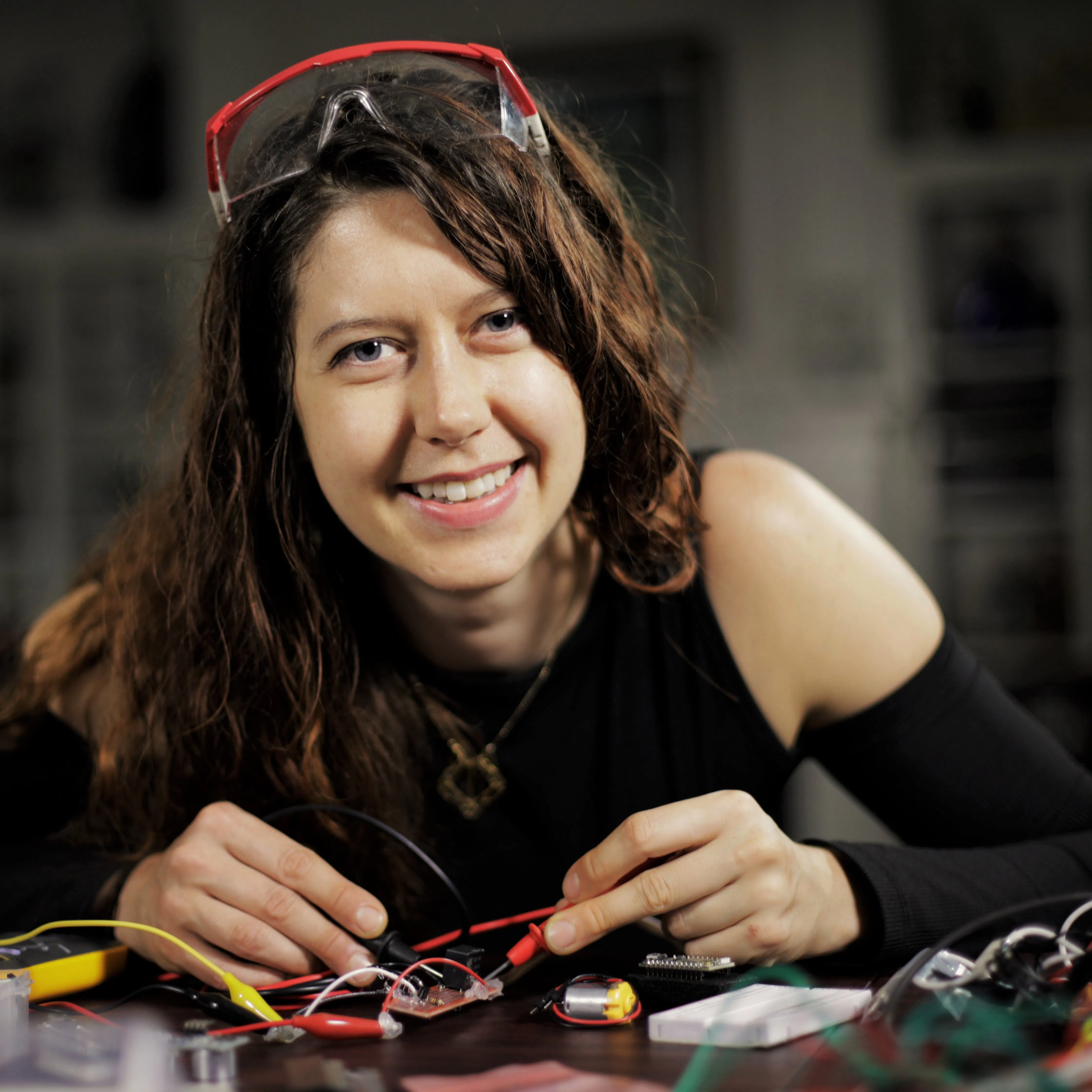Published

# Hacking STEM Heat Shield Simulation

Put on your aerospace engineering hat! Explore how different materials absorb and transmit heat to simulate space capsule re-entry to earth!

IntermediateProtip1.2 hours1,570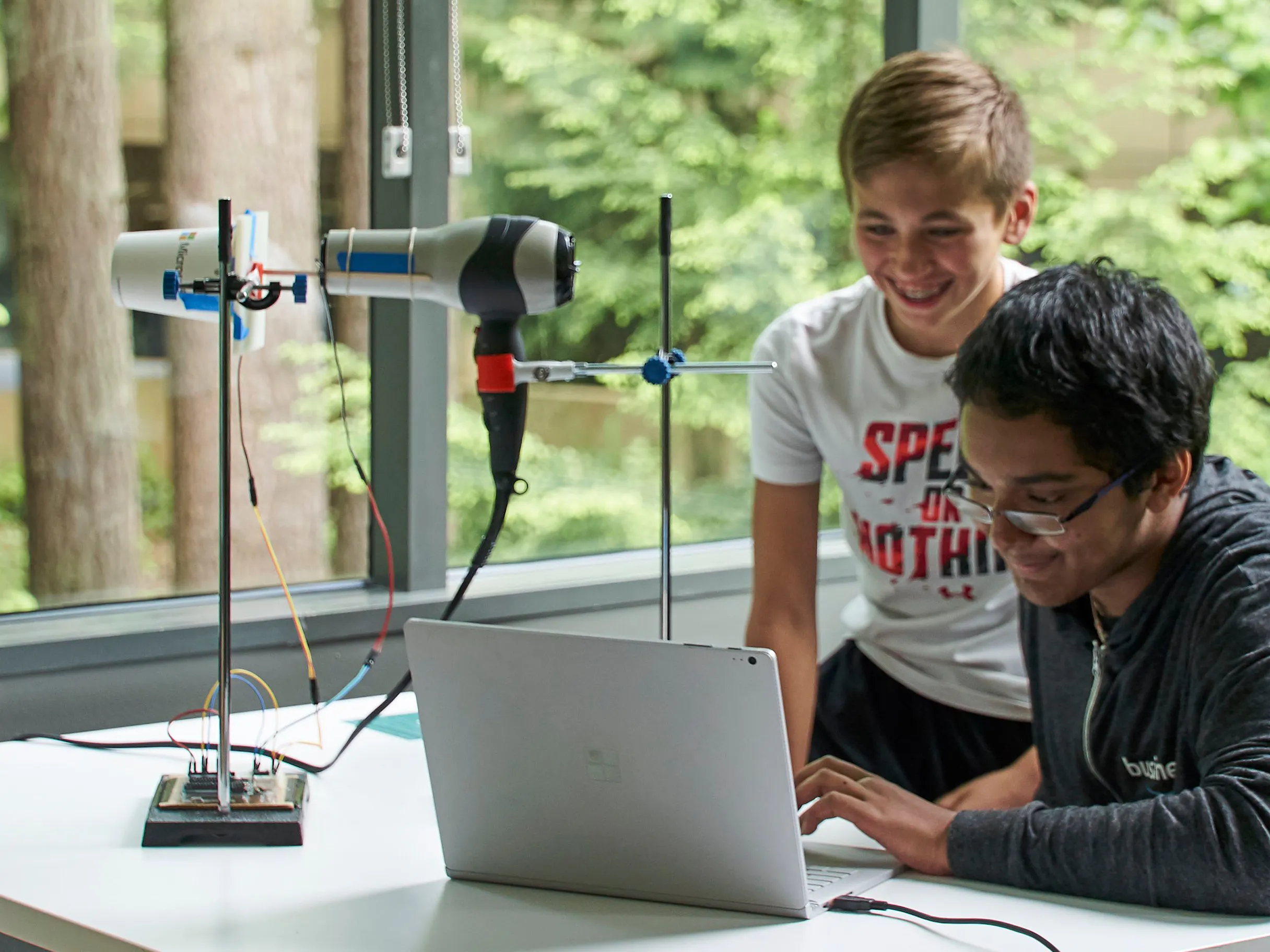## Things used in this project

### Hardware componentsArduino UNO & Genuino UNO
×1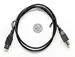USB-A to B Cable
×1Resistor 10k ohm
×2
 thermistor 10k
×2Jumper wires (generic)
×8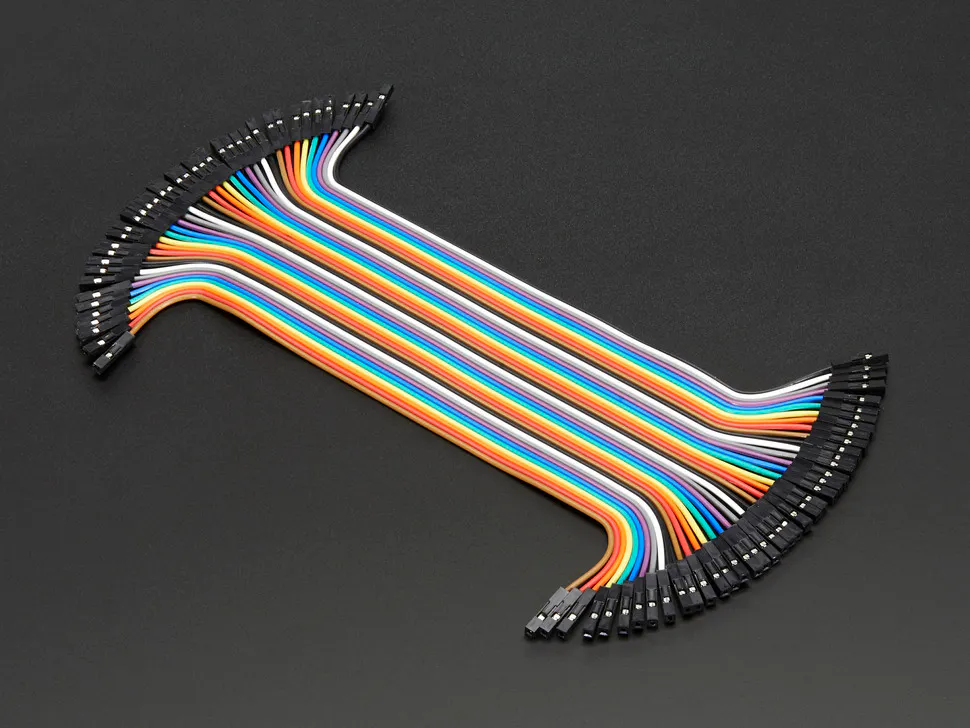Female/Female Jumper Wires
×4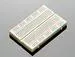×1
 rubber band
×2
 wooden stir stick
×1
 8oz paper cup
×1
 testing materials: 1 sheet of glass, nylon, aluminum, brass, and acrylic (10cm x 10cm)
×1

### Software apps and online servicesMicrosoft Data StreamerArduino IDE
 Microsoft Excel

### Hand tools and fabrication machines

 hair dryer
 ring stands with clamp (2)Hot glue gun (generic)
 metric ruler

## Schematics

### Arduino Heat Shield Electrical Diagram

A Fritzing-generated wiring diagram for connecting two thermistors to an Arduino Uno microcontroller.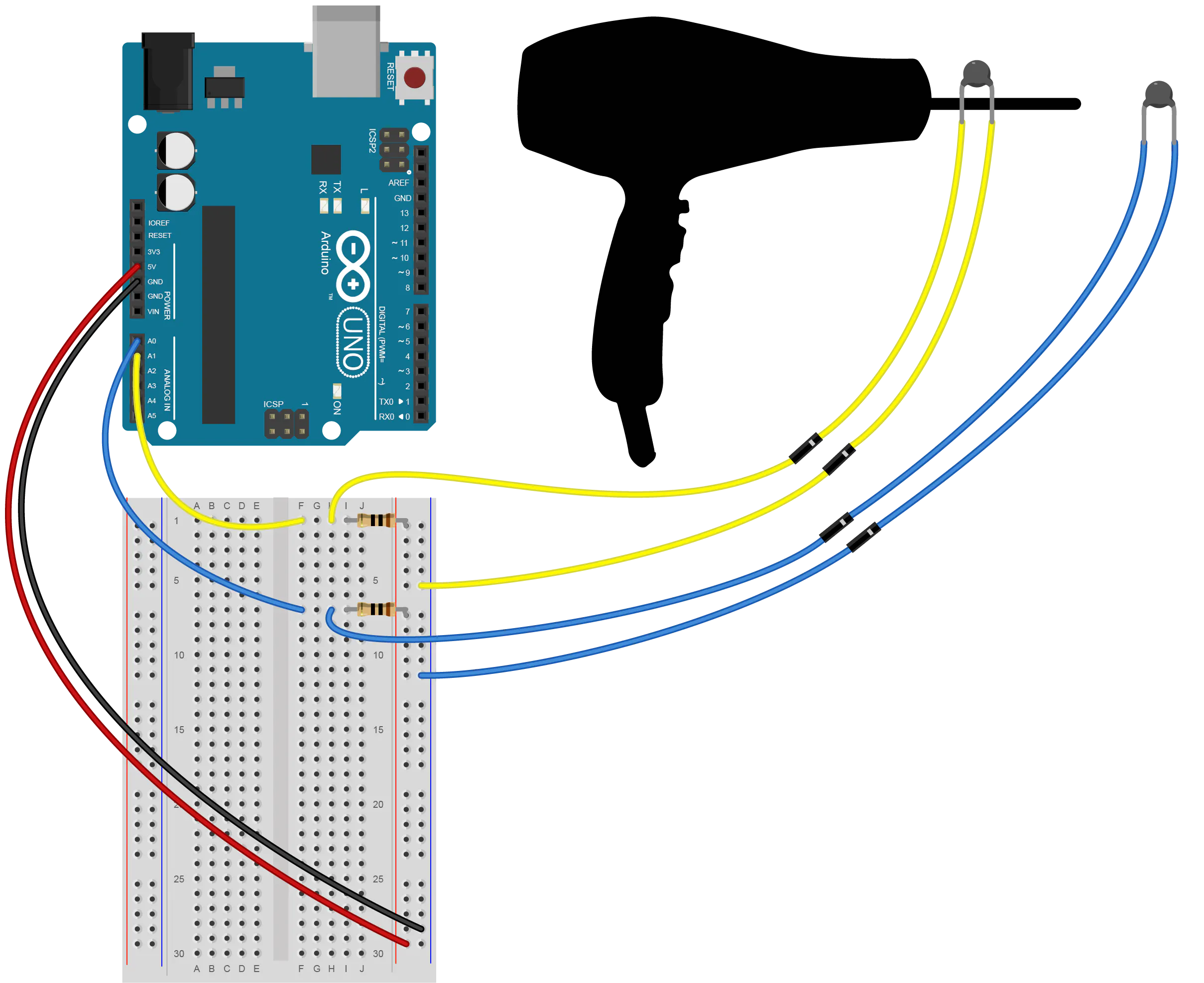## Code

### Thermistor

Arduino
This project reads the resistance of two thermistors and converts that value to temperature using the stienhart-hart formula. Each of the thermistors is set up with a voltage divider, so that we can use the voltage divider equation to accurately measure the resistance.
```// ----------------------------------------------------------------------------
// Thermistor.ino is for use with the Hacking STEM NASA Heat Shield lesson plan
// available from Microsoft Education Workshop at http://aka.ms/hackingSTEM
//
// This project uses an Arduino UNO microcontroller board. More information can
// be found by visiting the Arduino website:
// https://www.arduino.cc/en/main/arduinoBoardUno
//
// This project reads the resistance of two thermistors and converts that value
// to temperature using the stienhart-hart formula. Each of the thermistors is
// setup with a voltage divider, so that we can use the voltage divider
// equation to accurately measure the resistance.
//
// Pins:
// A0 Material Thermistor
// A1 Hair Dryer Thermistor
//
// Comments, contributions, suggestions, bug reports, and feature requests
// are welcome! For source code and bug reports see:
// http://github.com/[TODO: github path to Hacking STEM]
//
//
//
// ----------------------------------------------------------------------------

const int kMaterialThermPin = A0;
const int kHairDryerThermPin = A1;

const char kDelimiter = ',';    // Data Streamer expects a comma delimeter
const int kSerialInterval = 1000;   // Interval between serial writes
unsigned long serialPreviousTime; // Timestamp to track serial interval

void setup(void)
{
Serial.begin(9600);
}

void loop(void)
{
sendDataToSerial();
}

float getResistance(int pin)
{
const int kSeriesResistor = 10000;
//Read an analog pin and convert the value to a resistance value in ohms
float voltage = 1023 / reading - 1;
float resistance = kSeriesResistor / voltage;
return resistance;

}

{
// Resistance at 25 C
const int kThermistorNominal = 10000;
// Tempatrature for nominal resistance
const int kTemperatureNominal = 25;
// Beta coefficient of the thermistor
const int kBetaCoefficient = 3950;

// The stienhart-hart formula below is fairly complex for more info see:
// https://en.wikipedia.org/wiki/Thermistor
float value;
value = log(value);
value /= kBetaCoefficient;
value += 1.0 / (kTemperatureNominal + 273.15);
value = 1.0 / value;
value -= 273.15;
float temperature = value;
return temperature;
}

// OUTGOING SERIAL DATA PROCESSING CODE----------------------------------------
void sendDataToSerial()
{
// Enter into this only when serial interval has elapsed
if((millis() - serialPreviousTime) > kSerialInterval)
{
serialPreviousTime = millis(); // Reset serial interval timestamp
Serial.print(kDelimiter);

Serial.println(); // Add final line ending character only once
}
}
```

## Credits

### Hacking STEM

10 projects • 31 followers
Build affordable inquiry and project-based activities to visualize data across STEM curriculum.

### Jen Fox

17 projects • 48 followers
Dabbled in dark matter, settled into engineering w/ a blend of inventing and education! Founder/CEO of FoxBot Industries